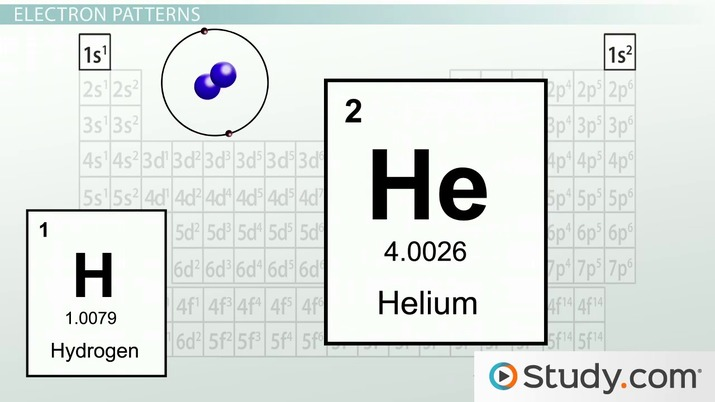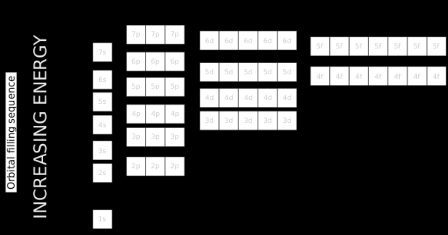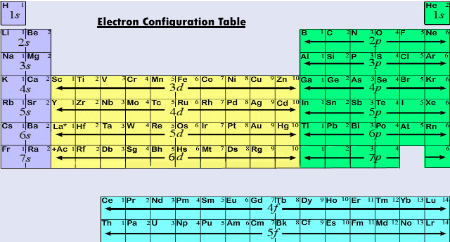# Electronic Configuration and Energy Levels

Kristin Born, Matthew Bergstresser
• Instructor
Kristin Born

Kristin has an M.S. in Chemistry and has taught many at many levels, including introductory and AP Chemistry.

• Expert Contributor
Matthew Bergstresser

Matthew has a Master of Arts degree in Physics Education. He has taught high school chemistry and physics for 14 years.

What is electron configuration? Learn how to do electron configuration. See the relationship between electron configuration and the energy levels of an atom. Updated: 08/13/2021

Show

## What is Electron Configuration?

Each atom is unique and has a set number of protons. This is called the atomic number. Each proton is balanced out by the opposite charge of an electron. But unlike protons, electrons do not inhabit the nucleus, rather they orbit around the protons and neutrons in specific patterns. Those patterns are then expressed by the electron configuration. Electron configuration is a numerical expression of a 3D space around an atom's nucleus.An error occurred trying to load this video.

Try refreshing the page, or contact customer support.

Coming up next: Four Quantum Numbers: Principal, Angular Momentum, Magnetic & Spin

### You're on a roll. Keep up the good work!

Replay
Your next lesson will play in 10 seconds
• 0:07 Electrons
• 1:20 Quantities of Electrons
• 2:47 Electron Patterns
• 6:36 Electron Configurations
• 9:20 Making Predictions
• 9:55 Lesson Summary
Save Save

Want to watch this again later?

Timeline
Autoplay
Autoplay
Speed Speed

## How Are Electrons Arranged in an Atom?

The Bohr model of the atom has the electrons orbiting around the nucleus like planets around the sun. This is a simplistic and flawed model of the quantum world. Electrons occupy a three-dimensional space around the atom, forming an "electron cloud" as opposed to a straight orbit. However, the Bohr model is still a good way to illustrate the atom and its parts. Electrons occupy orbitals or areas around the nucleus. Electrons can give off and absorb "specific" amounts of energy, or quanta (thus the birth of quantum mechanics) to move from one orbit to another. All the orbitals are layered around an atom, giving each orbital area a unique energy level, direction of spin, shape, and size.

## Quantum Numbers

### Quantum Number n, Principal Quantum Number

The letter n denotes what energy level the electron inhabits. Any nonzero integer is a possible value for n= 1, 2, 3, and so on. This denotes the shell the electron is in. Any element in row 1 will have electrons with the principal quantum number n=1. Row two elements have n=2, row three n=3, row four n=4, all the way to seven. Theoretically, there could be n=113, but that would require elements filling up all the previous n values.

### Quantum Number l, Orbital Angular Momentum

Angular momentum is a fancy way of describing the space an electron could occupy. If n is any nonzero integer, then its orbital angular momentum is signified by l=0 to n-1. The possible values for l are 0, 1, 2, etc. to infinity. The integers 0, 1, 2, and 3 indicate what subshell the electron is found in. s levels are denoted 0, p levels are 1, d levels are 2, and f levels are 3. For example, helium is in the first row, so n=1 and l=0. The s sublevel has one orbital. Every subshell orbital can hold a maximum of two electrons. These "shells" as they are called, are areas where the electron is most likely to be found, not a definite location. The s sublevel looks like a sphere, the p sublevel looks like two balloons tied together at the ends, the d sublevel is a combination of lobes and donuts, and f is a complicated multilobed balloon sculpture surrounding the nucleus.

### Quantum Number, Magnetic{eq}m_l {/eq}

The qunatum number denoted by {eq}m_l {/eq} describes the orientation and number of subshells within any given shell. The values for {eq}m_l {/eq} are dependent on the value of l, thus the subscript. Whatever value l has, {eq}m_l {/eq} can be -l to l. For example, if l=2, then {eq}m_l {/eq} values are -2, -1, 0, 1, 2. Therefore, for l=2, there can be 5 subshells, each with their own integer.

### Quantum number {eq}m_s {/eq}, Electron Spin

Electrons all have the same negative charge. The spin of each electron in a subshell will be opposite to the other. This is denoted as +1/2 or {eq}\uparrow {/eq} or -1/2 or {eq}\downarrow {/eq}. Unlike l which is dependent on n, or {eq}m_l {/eq} which is dependent on l, {eq}m_s {/eq} is not dependent on any other quantum number. The electron spin is either up or down, one of each in each subshell. The significance of the electron spin determines if the atom can generate a magnetic field.

### Shells and Subshells

Since electrons are physical things, they inhabit a physical place. These places, or shells, can be likened to a house. Each atom has its own "house" and the electrons of that atom occupy the rooms in this "house." How can the number of electrons an atom can hold be determined? That is where the quantum numbers come in.

If n=1, there is one floor or level. If n=1, then l=0, and 0 corresponds to sublevel s. The s sublevel is the simplest and the smallest, forming a spherical electron cloud around the nucleus. If one looks at the periodic table, one will see only two elements in row one. That is because there is only one sublevel in row one, only able to hold two electrons, one for hydrogen and two for helium. The four quantum numbers (n, l, {eq}m_l {/eq}, {eq}m_s {/eq}) for hydrogen's electron are (1,0,0,+1/2). Since helium has two electrons, their quantum numbers will be (1,0,0,+1/2) and (1,0,0,-1/2)

when n=2, then l= 0 and 1. In row two of the periodic table, there are two orbitals, 0=s, and 1=p. P sublevels can hold six electrons. Boron is the first element to have electrons that are a sublevel other than s. It has five electrons, so one is in a sublevel or subshell p. Let's write out all the atomic numbers for boron's five electrons:

• (1,0,0,+1/2)
• (1,0,0,-1/2)
• (2,0,0,+1/2
• (2,0,0,-1/2)
• (2,1,-1,+1/2)

Take a look at the last set of quantum numbers. This time the value for the magnetic number is -1. This number is dependent on l, and can be -1,0,1. Thus, p subshells can have six electrons. It has three orbitals, each holding two electrons. The {eq}m_l {/eq} can be chosen at random when there is only one electron to contend with in a particular orbital.

## Energy of Atomic Orbitals

Since electrons are always moving, they always have energy. The energy an electron needs to be in a shell or subshell increases with the distance from the atomic nucleus. Electrons in a 1s shell will have less energy than electrons in a 2s or 3s shell. Electrons with the same n number but different l numbers are a bit more complicated. s orbitals will have less energy than p or d or f subshells. p orbitals will have less than d or f, and so on.The electron configuration pattern is discernable when looking at a periodic table. The n=3 shell, or 3rd row, has 3s and 2p sublevels. Electrons will always fill sublevels according to the lowest energy first. There are some other rules that electrons generally follow as well.

## Filling of Atomic OrbitalsJust because electrons are home alone does not mean they do not follow the rules. There are rules for everything, this is physics.

### Aufbau Principle

The first rule is the Aufbau Principle. This states that electrons will fill lower energy levels before moving onto higher ones. This is why the elements in the middle of the periodic table, the transition elements, have 3d sublevels full before they fill the 4p sublevels. The 3d orbitals require less energy than the 4p. The order of filling for the sublevels would be 1s 2s 2p 3s 3p 4s 3d 4p 5s 4d 5p 6s 4f 5d 6p 7s 5f 6d 7p. If the amount of electrons is added, it would look like: {eq}1s^2 2s^2 2p^6 3s^2 3p^6 4s^2 3d^{10} 4p^6 5s^2 4d^{10} 5p^6 6s^2 4f^{14} 5d^{10} 6p^6 7s^2 5f^{14} 6d^{10} 7p^6 {/eq}

### Pauli Exclusion Principle

The Pauli Exclusion Principle states that the two electrons in an orbital have to have opposite spins and different quantum numbers. One will always be spin up (+1/2) and one will be spin down (-1/2). There are two ways of writing this out, one way is with the quantum numbers, and the other is the "box and arrow" method.

No two electrons of any element will have the same set of quantum numbers. Oxygen is the eighth element on the periodic table. It is in row two, so n=2. When n=2, l value can be 0, or 1. For l=0, m_l=0; l=1, then {eq}m_l {/eq}= -1, 0, 1. {eq}m_s {/eq} can only be +1/2 or -1/2. The last four electrons in oxygen will have the quantum numbers (2, 1, -1, +1/2), (2, 1, 0, +1/2), (2, 1, 1, +1/2), and (2, 1, -1, -1/2). This is a little hard to visualize so let's see the box and arrow method. Each box will represent a sublevel. The arrows inside, one up and one down, will represent the spin of the electron. For the last four electrons of oxygen-### Hund's Rule

Hund's Rule states that in sublevels with multiple orbitals (p, d, and f) each orbital will get one electron before they pair up. Using these rules, the electron configuration of any element can be theorized. Look at the box and arrow diagram for oxygen. There are electrons in all three p sublevels instead of two in l=-1 and l=0. The first three electrons will spread out, due to their like charge. The fourth electron will then fill in after.

To unlock this lesson you must be a Study.com Member.

• Activities
• FAQs

## Electron Configuration

Each atom has electrons in its electron cloud. The number of electrons in an atom's electron cloud is that element's atomic number. These electrons are arranged in specific energy levels surrounding the nucleus. In this activity, we are going to use the periodic table to determine the electron configuration of a few elements. You will need to have a periodic table for this activity.

### Labeling the Periodic Table

The first two columns on the left side of the periodic table are the s-block elements. The middle section up to group 12 is the d-block. The remaining groups comprise the p-block elements except for helium. It is in the s-block. We won't work with the f-block, which are the bottom two rows that are separated from the main two groups.

The rows tell us what energy level the electron is in. The group number within the block tells us how many electrons are in that subshell. We'll start at the element we are working with and work backward.

For example, lithium's electron configuration is 1s2 2s1. We start at lithium on the periodic table, and we see it is in the second row and the first column of the s-block, so it's electron configuration ends in 2s1. All inner orbitals are filled, which means 1s2% is complete. This gives us 2s1 1s2. Reversing this order gives us the correct electron configuration of 1s2 2s1.

### Electron Configuration Practice

1. Write the electron configuration for beryllium.
2. Write the electron configuration for sulfur.
3. Write the electron configuration for calcium.

1. 1s2 2s2
2. 1s2 2s2 2p6 3s2 3p4
3. 1s2 2s2 2p6 3s2 3p6 4s2

#### How many electrons are in each energy level?

S levels only hold two electrons. P levels can hold six. D levels hold 10 electrons, and F levels hold 14.

#### What are the 4 energy levels?

The four energy levels for electrons are s, p, d, and f. s level holds 2 electrons; p level holds 8 electrons; d level holds 10 electrons; f level holds 14 electrons.

#### How do you write the electron configuration of an element?

Electron configuration takes the total number of electrons and assigns them specified positions around the atom. Electron shells will fill in a certain order until all electrons have positions, starting with 1s, then 2s, then 2p, then 3s, 3p, 4s, 4d, 4p, and so on.

#### What is 1s 2s 2p 3s 3p?

This is shorthand electron configuration. S is an s level, P is a p level. S levels hold 2 electrons each. P holds eight electrons each. The large numbers in front tell which row of the periodic table is being discussed. The largest number in the configuration is always the outermost shell.

### Register to view this lesson

Are you a student or a teacher?